Courses
Courses for Kids
Free study material
Offline Centres
More

# NCERT Solutions for Class 6 Maths Chapter 10 - In HindiLast updated date: 29th Nov 2023
Total views: 429k
Views today: 10.29k

## NCERT Solutions for Class 6 Maths Chapter 10 Mensuration in Hindi PDF Download

Download the Class 6 Maths NCERT Solutions in Hindi medium and English medium as well offered by the leading e-learning platform Vedantu. If you are a student of Class 6, you have reached the right platform. The NCERT Solutions for Class 6 Maths in Hindi provided by us are designed in a simple, straightforward language, which are easy to memorise. You will also be able to download the PDF file for NCERT Solutions for Class 6 Maths in Hindi from our website at absolutely free of cost.

NCERT, which stands for The National Council of Educational Research and Training, is responsible for designing and publishing textbooks for all the classes and subjects. NCERT textbooks covered all the topics and are applicable to the Central Board of Secondary Education (CBSE) and various state boards.

We, at Vedantu, offer free NCERT Solutions in English medium and Hindi medium for all the classes as well. Created by subject matter experts, these NCERT Solutions in Hindi are very helpful to the students of all classes.

## NCERT Solution for Maths (Hindi) Chapter 10 - क्षेत्रमिति

### प्रश्नावली 10.1

1: नीचे दी हुई आकृतियों का परिमाप ज्ञात कीजिए:

(a)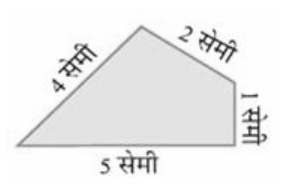उत्तर : परिमाप $=$चारों भुजाओ का योग
$=4\text{ }+\text{ }2\text{ }+\text{ }1\text{ }+\text{ }5\text{ }=\text{ }12$ सेमी

(b)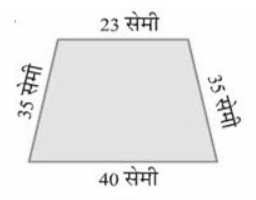उत्तर: परिमाप $=$ चारों भुजाओ का योग
= 35 + 2$=35\text{ }+\text{ }23\text{ }+\text{ }35\text{ }+\text{ }40\text{ }=\text{ }133$ सेमी

(c)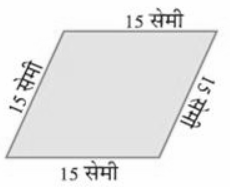उत्तर: परिमाप = चारों भुजाओ का योग

$=15\text{ }+\text{ }15\text{ }+\text{ }15\text{ }+\text{ }15\text{ }=\text{ }60$ सेमी

(d)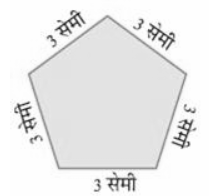उत्तर: परिमाप = सभी भुजाओं का योग $=~3\times 5=15~$ सेमी

(e)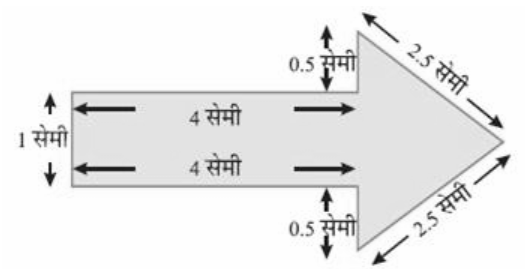उत्तर: परिमाप $=2\left( 1+4+0.5+2.5 \right)=2\times 8=16=2\left( 1+4+0.5+2.5 \right)=2\times 8=16~$ सेमी

(f)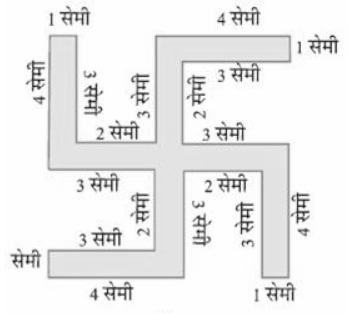उत्तर: परिमाप $=4\left( 3+4+1+3+2 \right)=4\times 13=52=4\left( 3+4+1+3+2 \right)=4\times 13=52~$ सेमी

2: 40 सेमी लंबाई और $\mathbf{10}$ सेमी चौड़ाई वाले एक बॉक्स के ढ़क्कन को चारों ओर से पूरी तरह एक टेप द्वारा बंद कर दिया जाता है। आवश्यक टेप की लंबाई ज्ञात कीजिए।

उत्तर: टेप की लंबाई $=$ बॉक्स का परिमाप

$=2$ (लंबाई +चौड़ाई )
$=2\left( 40+10 \right)$

$=2\times 50$

$=100$ सेमी

अतः, आवश्यक टेप की लंबाई $100$ सेमी होगी।

3. एक मेज़ की ऊपरी सतह की विमाएँ $\mathbf{2}$ मी $\mathbf{25}$ सेमी और $\mathbf{1}$ मी $\mathbf{50}$ सेमी हैं। मेज़ की ऊपरी सतह का परिमाप ज्ञात कीजिए।

उत्तर: आयत का परिमाप $=2$ (लंबाई $+$ चौड़ाई)
$=2\left( 2.25+1.50 \right)$

$=2\left( 2.25+1.50 \right)$

$=7.50$ मी

अतः, मेज़ की ऊपरी सतह का परिमाप $7.50$ मी है।

4. $\mathbf{32}$ सेमी लंबाई और $\mathbf{21}$ सेमी चौड़ाई वाले एक फ़ोटो को लकड़ी की पट्टी से फ्रेम करना है। आवश्यक लकड़ी की पट्टी की लंबाई ज्ञात कीजिए।

उत्तर: लकड़ी की पट्टी की लंबाई = फोटो फ्रेम का परिमाप

आयत का परिमाप $=2$ (लंबाई $+$ चौड़ाई)

=2(32+21)

$=2\times 53$

$=106$ सेमी

अतः, आवश्यक लकड़ी की पट्टी की लंबाई $106$ सेमी होगी।

5. एक आयताकार भूखंड की लंबाई और चौड़ाई क्रमश: $\mathbf{0}.\mathbf{7}$ किमी और $\mathbf{0}.\mathbf{5}$ किमी है। इसके चारों ओर एक तार से $\mathbf{4}$ पंक्तियों में बाड़ लगाई जानी है। आवश्यक तार की लंबाई ज्ञात कीजिए।

उत्तर: तार की एक पंक्ति की लंबाई $=$ आयताकार भूखंड का परिमाप
$=\text{ }2$ (लंबाई + चौड़ाई)

= 2(0.7 + 0.5)

$= 2 \times 1.2$

$=2.4$ किमी

इसीलिए, तार की आवश्यक लंबाई $=4\times 2.4=9.6$ सेमी है।

6. निम्न आकृतियों में प्रत्येक का परिमाप ज्ञात कीजिए:

1. एक त्रिभुज जिसकी भुजाएँ $\mathbf{3}$ सेमी, $\mathbf{4}$ सेमी और $\mathbf{5}$ सेमी हैं।

उत्तर: परिमाप $=3\text{ }+\text{ }4\text{ }+\text{ }5\text{ }=\text{ }12$ सेमी

1. एक समबाहु त्रिभुज जिसकी एक भुजा की लंबाई $\mathbf{9}$ सेमी है।

उत्तर: समबाहु त्रिभुज का परिमाप $=3\times$ एक भुजा की लंबाई

$=3\times 9$

$=27~$ सेमी

1. एक समद्विबाहु त्रिभुज जिसकी प्रत्येक समान भुजाएँ $\mathbf{8}$ सेमी की हो और तीसरी भुजा $\mathbf{6}$ सेमी हो।

उत्तर: समद्विबाहु त्रिभुज का  परिमाप $=$ सभी भुजाओ का योग

$=8+8+6$

$=22~$ सेमी

7. एक त्रिभुज का परिमाप ज्ञात कीजिए जिसकी भुजाएँ $\mathbf{10}$ सेमी, $\mathbf{14}$ सेमी और $\mathbf{15}$ सेमी हैं।

उत्तर: त्रिभुज का परिमाप $=$ भुजाओ का योग

$=10\text{ }+\text{ }14\text{ }+\text{ }15$

$=\text{ }39$ सेमी

8. एक सम षट्भुज का परिमाप ज्ञात कीजिए, जिसकी प्रत्येक भुजा की माप $\mathbf{8}$ मी है।

उत्तर: सम षटभुज का परिमाप $=6\times$ एक भुजा की लंबाई

$=6\times 8$

$=48~$ मी

9. एक वर्ग की भुजा ज्ञात कीजिए, जिसका परिमाप $\mathbf{20}$ मीटर है।

उत्तर: हम जानते हैं कि वर्ग का परिमाप $=4\text{ }\times$भुजा

इसलिए, भुजा $=$ परिमाप $\div \text{ }4$

$=\text{ }\frac{20}{4}\text{ }$

$=\text{ }5$ मी

10. एक सम पंचभुज का परिमाप $\mathbf{100}$ सेमी है। प्रत्येक भुजा की लंबाई ज्ञात कीजिए।

उत्तर: सम पंचभुज की भुजा $=$ परिमाप $\div \text{ }5$

$=\text{ }\frac{100}{5}\text{ }$

$=\text{ }20$ सेमी

11. एक धागे का टुकड़ा $\mathbf{30}$ सेमी लंबाई का है। प्रत्येक भुजा की लंबाई क्या होगी, यदि धागे से बनाया जाता है।

1. एक वर्ग?

उत्तर: वर्ग का परिमाप $=4\times$ वर्ग की एक भुजा की लंबाई

वर्ग की भुजा $=$ परिमाप $\div \text{ }4$
$=\text{ }\frac{30}{4}\text{ }$

$=\text{ }7.5$ सेमी

1. एक समबाहु त्रिभुज?

उत्तर: समबाहु त्रिभुज की भुजा $=$ परिमाप $\div \text{ }3$

$=\text{ }\frac{30}{3}\text{ }=\text{ }10$ सेमी

1. एक सम षट्भुज?

उत्तर: सम षटभुज की भुजा $=$ परिमाप $\div \text{ }6$

= 30 ÷ 6 = 5 सेमी

12. एक त्रिभुज की दो भुजाएँ $\mathbf{12}$ सेमी और 14 सेमी हैं। इस त्रिभुज का परिमाप $\mathbf{36}$ सेमी है। इस त्रिभुज की एक भुजा की लंबाई क्या होगी?

उत्तर: त्रिभुज का परिमाप $=$ तीनों भुजाओं का योग
या, $36\text{ }=\text{ }12\text{ }+\text{ }14\text{ }+$ तीसरी भुजा
या, तीसरी भुजा $=36\text{ }\text{ }\left( 12\text{ }+\text{ }14 \right)\text{ }=\text{ }36\text{ }\text{ }26\text{ }=\text{ }10$ सेमी

अतः, त्रिभुज की तीसरी भुजा $10$ सेमी होगी।

13. $\mathbf{250}$ मी भुजा वाले वर्गाकार बगीचे के चारों ओर बाड़ लगाने का व्यय ₨ $\mathbf{20}$ प्रति मीटर की दर से ज्ञात कीजिए।

उत्तर: वर्ग का परिमाप $=$ भुजा $\times \text{ }4$
$=\text{ }250\text{ }\times \text{ }4\text{ }=\text{ }1000$ मी
खर्च $=$दर $\times$ परिमाप
$=\text{ }20\text{ }\times \text{ }1000\text{ }=\text{ }20000$ रु.

अतः व्यय ₨ $\mathbf{20}$ प्रति मीटर की दर से $2000$ रु. होगा।

14. एक आयताकार बगीचा जिसकी लंबाई $\mathbf{175}$ मी और चौड़ाई $\mathbf{125}$ मी है, के चारों ओर $Rs.\mathbf{12}$ प्रति मीटर की दर से बाड़ लगाने का खर्च ज्ञात कीजिए।

उत्तर: आयत का परिमाप $=2$ (लंबाई +चौड़ाई)
$=\text{ }2\text{ }\left( 175\text{ }+\text{ }125 \right)\text{ }=\text{ }2\text{ }\times \text{ }300\text{ }=\text{ }600$मी
खर्च = दर × परिमाप
$=\text{ }12\text{ }\times \text{ }600\text{ }=\text{ }7200$रु

अतः ,चारों ओर $Rs.\mathbf{12}$ प्रति मीटर की दर से बाड़ लगाने का खर्च $7200$ रु. है।

15: स्वीटी $\mathbf{75}$ मीटर भुजा वाले वर्ग के चारों ओर दौड़ती है और बुलबुल $\mathbf{60}$ मी लंबाई और $\mathbf{45}$ मी चौड़ाई वाले आयत के चारों ओर दौड़ती है। कौन कम दूरी तय करती है?

उत्तर: वर्ग का परिमाप $=$ भुजा $\times \text{ }4$

$=\text{ }75\text{ }\times \text{ }4$

$=\text{ }300$ मी

अतः, स्वीटी द्वारा तय की गई दूरी $300$ मी है।

आयत का परिमाप $=2$ (लंबाई $+$ चौड़ाई)
$=\text{ }2\text{ }\left( 60\text{ }+\text{ }45 \right)$

$=\text{ }2\text{ }\times \text{ }105$

$=\text{ }210$ मी

अतः, बुलबुल द्वार तय दूरी $210$ मी है।
इस प्रकार यह स्पष्ट है कि बुलबुल कम दूरी तय करती है।

16. निम्न प्रत्येक आकृति का परिमाप ज्ञात कीजिए। आप उत्तर से क्या निष्कर्ष निकालते हैं?

(a)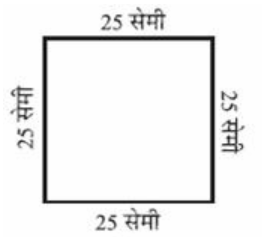उत्तर: वर्ग का परिमाप $=$ भुजा $\times \text{ }4$
$=\text{ }25\text{ }\times \text{ }4\text{ }=\text{ }100$ सेमी

(b)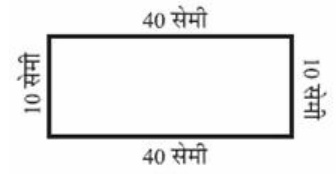उत्तर: आयत का परिमाप $=$$2$ (लंबाई $+$ चौड़ाई)
$=\text{ }2\text{ }\left( 30\text{ }+\text{ }20 \right)$

$=\text{ }2\text{ }\times \text{ }50$

$=\text{ }100$ सेमी

(c)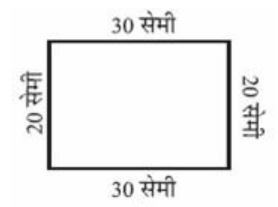उत्तर: आयत का परिमाप $=2$ (लंबाई $+$ चौड़ाई)
$=\text{ }2\text{ }\left( 40\text{ }+\text{ }10 \right)$

$=\text{ }2\text{ }\times \text{ }50$

$=\text{ }100$ सेमी

(d)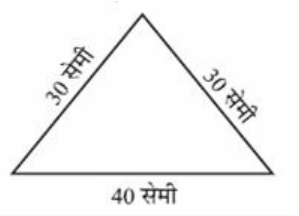उत्तर:त्रिभुज का परिमाप $=$ भुजाओं का योग
$=\text{ }30\text{ }+\text{ }30\text{ }+\text{ }40$

$=\text{ }100$ सेमी
(सभी आकृतियों के परिमाप बराबर हैं।)

17. अवनीत $\mathbf{9}$ वर्गाकार टाइल खरीदता है, जिसकी प्रत्येक भुजाएँ $\mathbf{1}/\mathbf{2}$ मीटर है और वह इन टाइलों को एक वर्ग के रूप में रखता है।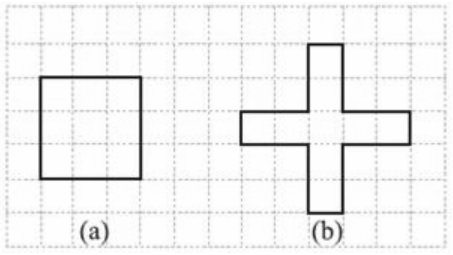1. नए वर्ग का परिमाप क्या है?

उत्तर: वर्ग की परिमाप $=4\times$ एक भुजा की लंबाई

$=4\text{ }\times ~1.5$

$=6$ मी

1. शैरी को उसके टाइलों को रखने का तरीका पसंद नहीं आता है। वह इन टाइलों को एक क्रास के रूप में रखवाती है। इस व्यवस्था का परिमाप क्या होगा?

उत्तर: क्रॉस का परिमाप $=4\text{ }\times ~\left( \frac{1}{2}+1+1 \right)$

$=\text{ }4\text{ }\times ~\frac{5}{2}$

$=\text{ }10$ मी

1.  किसका परिमाप अधिक है?

उत्तर: क्रॉस का परिमाप अधिक है।

1. अवनीत सोचता है कि, क्या कोई ऐसा भी तरीका है जिससे इनसे भी बड़ा परिमाप प्राप्त किया जा सकता हो? क्या आप ऐसा करने का कोई सुझाव दे सकते हैं?

( टाइलें किनारे से आपस में मिली हुई हों और वह टूटी हुई नहीं हों )।

उत्तर: ऐसा संभव नहीं है।

### प्रश्नावली 10.2

1. निम्नलिखित आकृतियों के क्षेत्रफल ज्ञात कीजिए: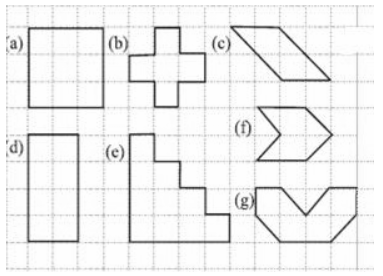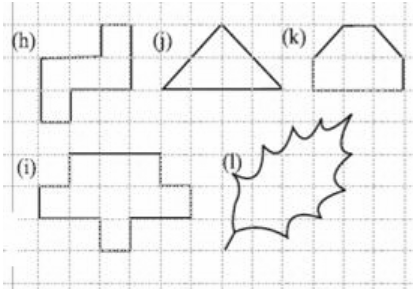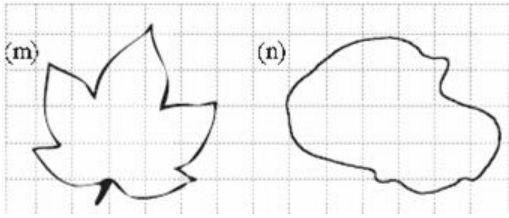उत्तर:

(a) पूरे घिरे वर्गों की संख्या $=9$

क्षेत्रफल $=9\times 1=9$ वर्ग इकाई

(b) पूरे घिरे वर्गों की संख्या $=5$

क्षेत्रफल $=5\times 1=5$ वर्ग इकाई

(c) पूरे घिरे वर्गों की संख्या $=2$

आधे घिरे वर्गों की संख्या $=4$

क्षेत्रफल = $2 + \frac{1}{2}\left( 4 \right) = 4$ वर्ग इकाई

(d) पूरे घिरे वर्गों की संख्या $=8$

क्षेत्रफल $=8\times 1=8$ वर्ग इकाई

(e) पूरे घिरे वर्गों की संख्या $=10$

क्षेत्रफल $=10\times 1=10$ वर्ग इकाई

(f) पूरे घिरे वर्गों की संख्या $=2$

आधे घिरे वर्गों की संख्या $=4$

क्षेत्रफल = $2 + \frac{1}{2}\left( 4 \right) = 4$ वर्ग इकाई

(g) पूरे घिरे वर्गों की संख्या $=4$

आधे घिरे वर्गों की संख्या $=4$

क्षेत्रफल = $4 + \frac{1}{2}\left( 4 \right) = 6$ वर्ग इकाई

(h) पूरे घिरे वर्गों की संख्या $=5$

क्षेत्रफल $=5\times 1=5$ वर्ग इकाई

(i) पूरे घिरे वर्ग की संख्या $=9$

क्षेत्रफल $=9\times 1=9$ वर्ग इकाई

(j) पूरे घिरे वर्गों की संख्या $=2$

आधे घिरे वर्गों की संख्या $=4$

क्षेत्रफल = $2 + \frac{1}{2}\left( 4 \right) = 4$ वर्ग इकाई

(k) पूरे घिरे वर्गों की संख्या $=4$

आधे घिरे वर्गों की संख्या $=2$

क्षेत्रफल = $4 + \frac{1}{2}\left( 2 \right) = 5$ वर्ग इकाई

(l) पूरे घिरे वर्गों की संख्या $=3$

आधे घिरे वर्गों की संख्या $=6$

कुल क्षेत्रफल  $3 + \frac{1}{2}\left( 6 \right) = 6$ वर्ग इकाई

(m) पूरे घिरे वर्गों की संख्या $=7$

आधे घिरे वर्गों की संख्या $=10$

कुल क्षेत्रफल  $7 + \frac{1}{2}\left( 10 \right) = 12$ वर्ग इकाई

(n) पूरे घिरे वर्गों की संख्या $=13$

आधे घिरे वर्गों की संख्या $=10$

कुल क्षेत्रफल $13 + \frac{1}{2}\left( {10} \right) = 18$ वर्ग इकाई

### प्रश्नावली 10.3

1: उन आयातों का क्षेत्रफल ज्ञात कीजिए जिनकी भुजाएँ नीचे दी गई हैं:

1. $\mathbf{3}$ सेमी और $\mathbf{4}$ सेमी

उत्तर: क्षेत्रफल $=$ लंबाई $\times$ चौड़ाई

$=\text{ }3\text{ }\times \text{ }4\text{ }=\text{ }12$ वर्ग सेमी

1. $\mathbf{12}$ मी और $\mathbf{21}$ मी

उत्तर: क्षेत्रफल $=$ लंबाई $\times$ चौड़ाई

$=\text{ }12\text{ }\times \text{ }21\text{ }=\text{ }252$ वर्ग मी

1. $\mathbf{2}$ किमी और $\mathbf{3}$ किमी

उत्तर: क्षेत्रफल $=$ लंबाई $\times$ चौड़ाई

$=\text{ }2\text{ }\times \text{ }3\text{ }=\text{ }6$ वर्ग किमी

1. $\mathbf{2}$ मी और $\mathbf{70}$ सेमी

उत्तर: क्षेत्रफल $=$ लंबाई $\times$ चौड़ाई

$=\text{ }2\text{ }\times \text{ }0.7\text{ }=\text{ }1.4$ वर्ग मी

2. उन वर्गों का क्षेत्रफल ज्ञात कीजिए जिनकी भुजाएँ निम्नलिखित हैं:

1. $\mathbf{10}$ सेमी

उत्तर: वर्ग का क्षेत्रफल $=$ भुजा $\times$ भुजा
$=\text{ }10\text{ }\times \text{ }10\text{ }=\text{ }100$ वर्ग सेमी

1. $\mathbf{14}$ सेमी

उत्तर: वर्ग का क्षेत्रफल $=$ भुजा $\times$ भुजा

$=14\text{ }\times \text{ }14\text{ }=\text{ }196$ = 196 वर्ग सेमी

1. $\mathbf{5}$ मी

उत्तर: वर्ग का क्षेत्रफल $=$ भुजा $\times$ भुजा

$=\text{ }5\text{ }\times \text{ }5\text{ }=\text{ }25$ वर्ग मी

3. तीन आयतों की विमाएँ निम्नलिखित हैं:

1. $\mathbf{9}$ मी और $\mathbf{6}$ मी

2. $\mathbf{3}$ मी और $\mathbf{17}$ मी

3. $\mathbf{4}$ मी और $\mathbf{14}$ मी

इनमें से किसका क्षेत्रफल सबसे अधिक है और किसका सबसे कम?

उत्तर: (सूत्र –आयात का क्षेत्रफल $=$ लंबाई $\times$ चौड़ाई)

1. पहले आयत का क्षेत्रफल $=9\text{ }\times \text{ }6\text{ }=\text{ }54$ वर्ग मी

2. दूसरे आयत का क्षेत्रफल $=3\text{ }\times \text{ }17\text{ }=\text{ }51$ वर्ग मी

3. तीसरे आयत का क्षेत्रफल $=4\text{ }\times \text{ }14\text{ }=\text{ }56$ वर्ग मी

अतः, दूसरे (b) आयत का क्षेत्रफल सबसे कम और तीसरे (c) आयत का क्षेत्रफल सबसे अधिक है।

4. $\mathbf{50}$ मी लंबाई वाले एक आयताकार बगीचे का क्षेत्रफल $\mathbf{300}$ वर्ग मीटर है। बगीचे की चौड़ाई ज्ञात कीजिए?

उत्तर: हम जानते हैं की आयात का क्षेत्रफल $=$ लंबाई $\times$ चौड़ाई
इसलिए, चौड़ाई $=$ क्षेत्रफल $/$ लंबाई
$=\frac{300}{50}\text{ }$

$=\text{ }6$मी

5. $\mathbf{500}$ मी लंबाई और $\mathbf{200}$ मी चौड़ाई वाले एक आयताकार भूखंड पर $Rs.\mathbf{8}$ प्रति $\mathbf{100}$ वर्ग मीटर की दर से टाइल लगाने का खर्च ज्ञात कीजिए?

उत्तर: आयात का क्षेत्रफल $=$ लंबाई $\times$ चौड़ाई
$=\text{ }500\text{ }\times \text{ }200$

$=\text{ }100000$वर्ग मी

प्रश्ननुसार, $100$ वर्ग मीटर का खर्च $=8$ रु

इसलिए, $1$ वर्ग मी का खर्च $=\frac{8}{100}=\text{ }0.08$ रु.

इसलिए, पूरे भूखंड पर टाइल लगाने का खर्च $=100000\text{ }\times \text{ }0.08\text{ }=\text{ }8000$ रु.

6. एक मेज़ की ऊपरी पृष्ठ की माप $\mathbf{2}$ मी $\times \text{ }\mathbf{1}$ मी $\mathbf{50}$ सेमी है। मेज़ का क्षेत्रफल वर्ग मीटर में ज्ञात कीजिए।

उत्तर: मेज की लंबाई = 2मी

मेज की चौड़ाई =$1$मी$50$सेमी$=1.50$मी

मेज का  क्षेत्रफल $=2\text{ }\times \text{ }1.5\text{ }=\text{ }5$वर्ग मी

7. एक कमरे की लंबाई $\mathbf{4}$ मी तथा चौड़ाई $\mathbf{3}$ मी $\mathbf{50}$ सेमी है। कमरे के फर्श को ढकने के लिए कितने वर्ग मीटर गलीचे की आवश्यकता होगी?

उत्तर: कमरे की लंबाई $=4$ मी

कमरे की चौड़ाई$=$ $\mathbf{3}$मी $\mathbf{50}$सेमी$=3.50$मी

कमरे का  क्षेत्रफल $=4\text{ }\times \text{ }3.5\text{ }=\text{ }14$वर्ग मी

8. एक फर्श की लंबाई $\mathbf{5}$ मी और चौड़ाई $\mathbf{4}$ मी है। $\mathbf{3}$ मी भुजा वाले एक वर्गाकार गलीचे को फर्श पर बिछाया गया है। फर्श के उस भाग का क्षेत्रफल ज्ञात किजिए जिस पर गलीचा नहीं बिछा है।

उत्तर: फर्श का क्षेत्रफल $=$ लंबाई $\times$ चौड़ाई

फर्श का क्षेत्रफल $=5\text{ }\times \text{ }4\text{ }=\text{ }20$ वर्ग मी

वर्गाकार गलीचे का क्षेत्रफल$=$भुजा $\times$ भुजा

गलीचे का क्षेत्रफल$=3\text{ }\times \text{ }3\text{ }=\text{ }9$ वर्ग मी

शेष भाग का क्षेत्रफल $=20\text{ }\text{ }9\text{ }=\text{ }11$ वर्ग मी

9. $5$ मी लंबाई तथा $\mathbf{4}$ मी चौड़ाई वाले एक आयताकार भूखंड पर $\mathbf{1}$ मी भुजा वाली वर्गाकार फूलों की $\mathbf{5}$ क्यारियाँ बनाई जाती हैं। भूखंड के शेष भाग का क्षेत्रफल ज्ञात कीजिए?

उत्तर: भूखंड का क्षेत्रफल $=$ लंबाई $\times$ चौड़ाई

भूखंड का क्षेत्रफल $=5\times \text{ }4=20$ वर्ग मी

वर्गाकार क्यारी का क्षेत्रफल $=1\text{ }\times 1=\text{ }1$ वर्ग मी

$5$ क्यारियों का क्षेत्रफल $=5\text{ }\times \text{ }1\text{ }=\text{ 5}$ वर्ग मी

शेष भूखंड का क्षेत्रफल $=20\text{ }\text{ 5 }=\text{ }15$ वर्ग मी

10. निम्नलिखित आकृतियों को आयातों में तोड़िए। इनका क्षेत्रफल ज्ञात कीजिए ( भुजाओं की माप सेमी में दी गई है )?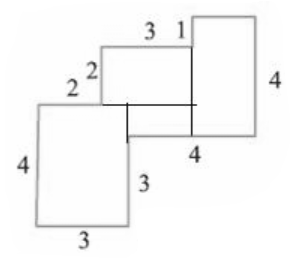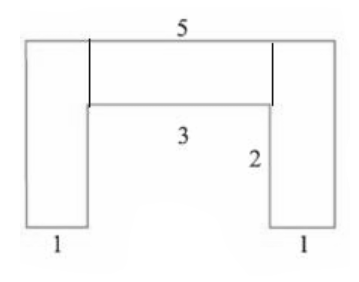उत्तर: (a)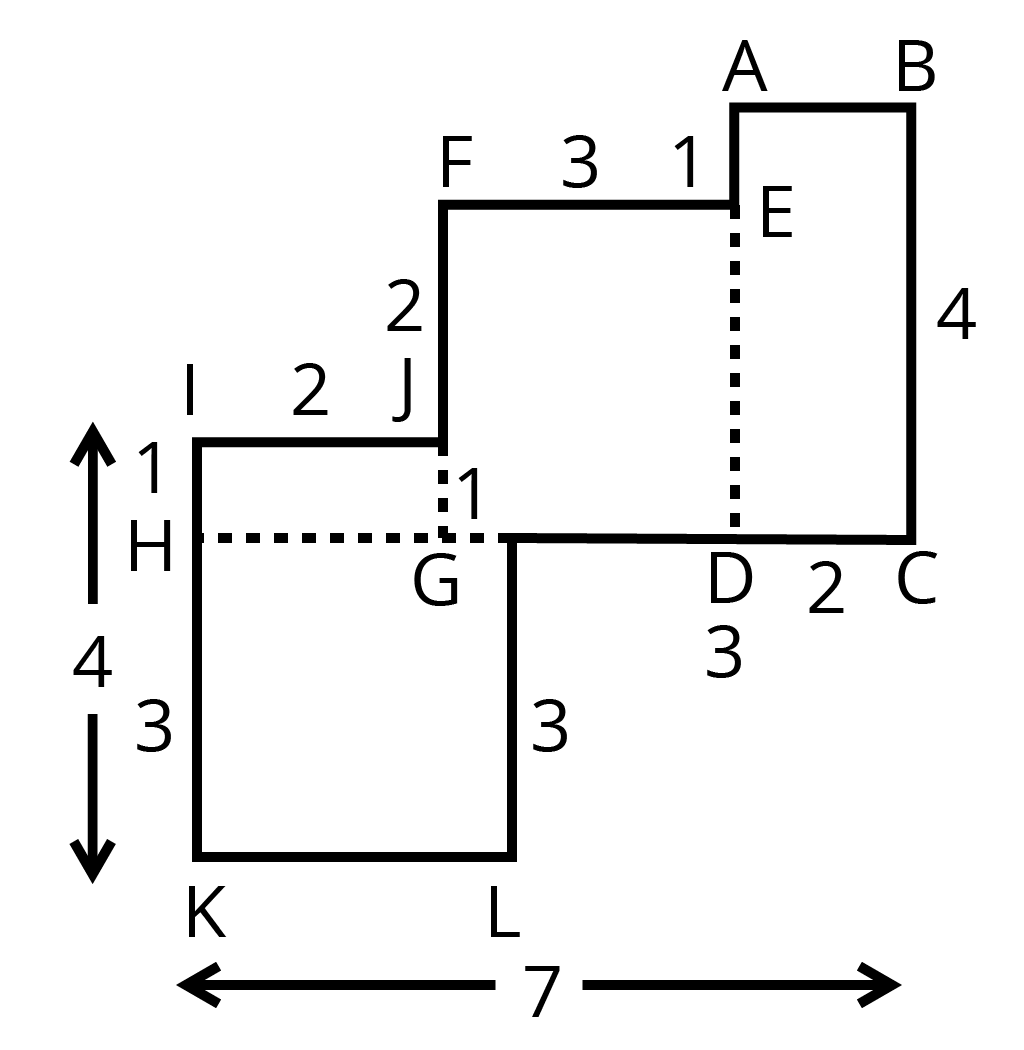$HKLM$ का क्षेत्रफल$=3\times 3=9$ वर्ग सेमी

$IJGH$ का क्षेत्रफल$=1\times 2=2$ वर्ग सेमी

$FEDG$ का क्षेत्रफल $=3\times 3=9$ वर्ग सेमी

$ABCD$ का क्षेत्रफल $=2\times 4=8$ वर्ग सेमी

आकृति का कुल क्षेत्रफल $=9+2+9+8=28$ वर्ग सेमी
उत्तर: (b)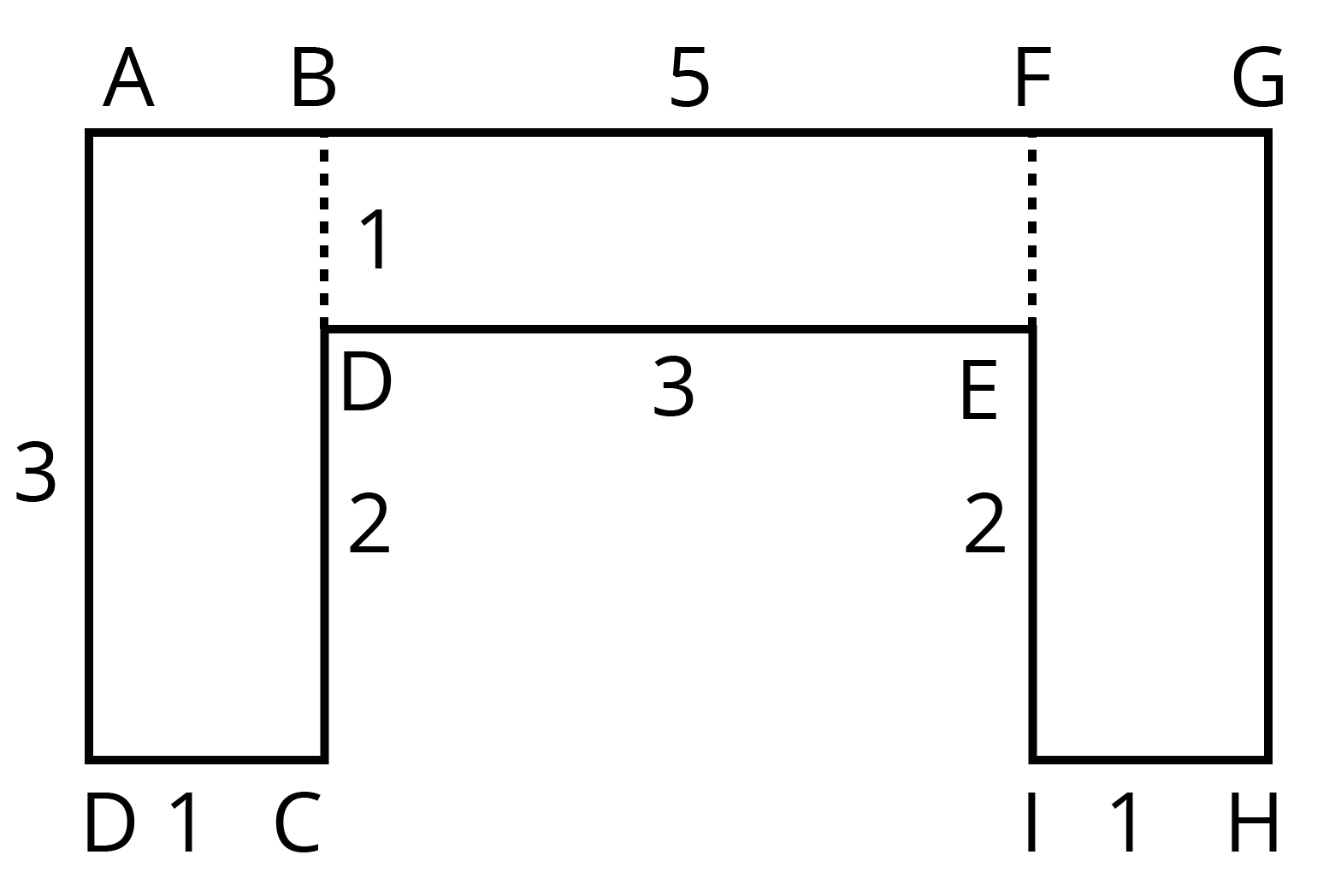$ABCD$ का क्षेत्रफल$=3\times 1=3$ वर्ग सेमी

$BDEF$ का क्षेत्रफल$=3\times 1=3$ वर्ग सेमी

$FGHI$ का क्षेत्रफल $=3\times 1=3$ वर्ग सेमी

आकृति का कुल क्षेत्रफल $=3+3+3=9$ वर्ग सेमी

11. निम्नलिखित आकृतियों को आयातों में तोड़िए। इनका क्षेत्रफल ज्ञात कीजिए ( भुजाओं की माप सेमी में दी गई है )?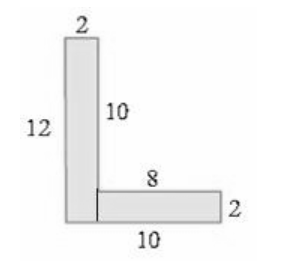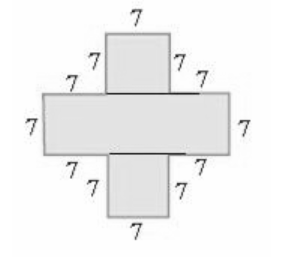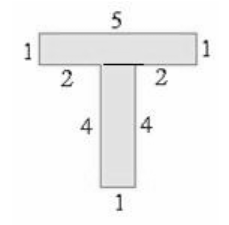उत्तर: (a)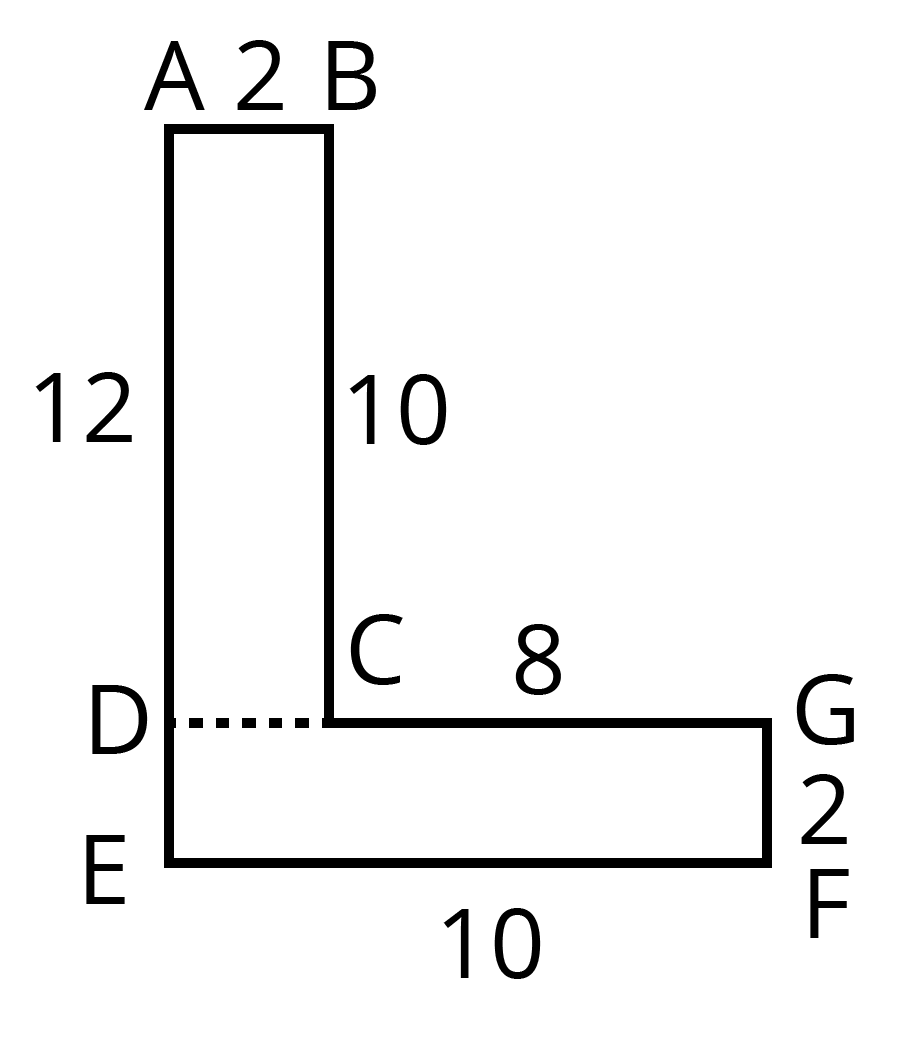$ABCD$ का क्षेत्रफल$=2\times 10=20$ वर्ग सेमी

$DFEG$ का क्षेत्रफल$=10\times 2=20$ वर्ग सेमी

आकृति का कुल क्षेत्रफल $=20+20=40$ वर्ग सेमी

उत्तर: (b)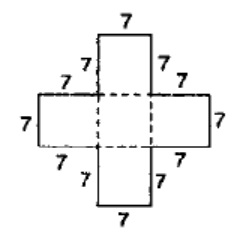यहाँ कुल सात वर्ग है जहां प्रत्येक की भुजा $7$ सेमी है।

एक वर्ग का क्षेत्रफल $=7\text{ }\times \text{ }7=49$ वर्ग सेमी

5 वर्गो का कुल क्षेत्रफल $=49\times 5=245$ वर्ग सेमी

उत्तर: (e)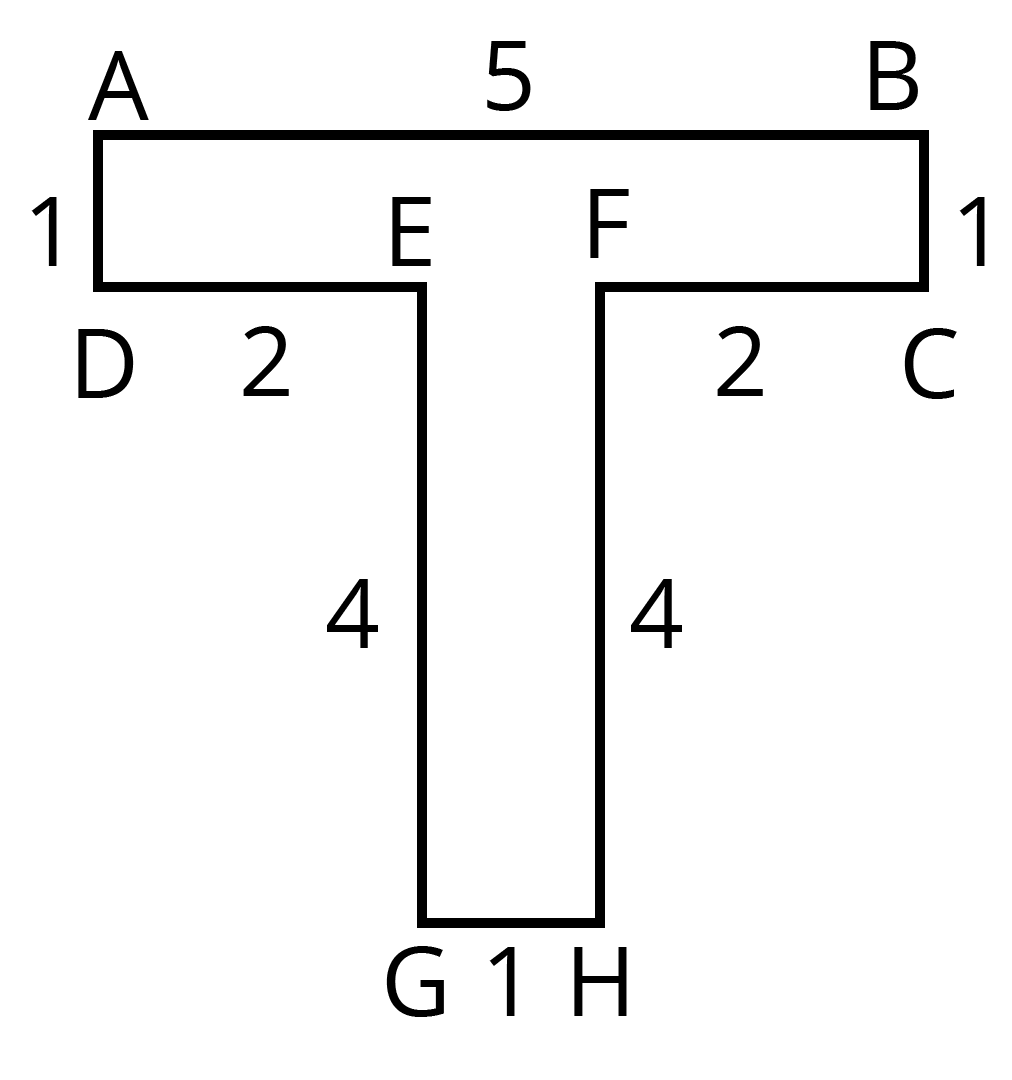$ABCD$ का क्षेत्रफल$=5\times 1=5$ वर्ग सेमी

$EFGH$ का क्षेत्रफल$=4\times 1=4$ वर्ग सेमी

आकृति का कुल क्षेत्रफल $=5+4=9$ वर्ग सेमी

12. एक टाइल की माप $\mathbf{5}$ सेमी $\times \text{ }\mathbf{12}$ सेमी है। एक क्षेत्र को पूर्णतः ढकने के लिए, ऐसी कितनी टाइलों की आवश्यकता होगी, जिसकी लंबाई और चौड़ाई क्रमश:

1. $\mathbf{144}$ और $\mathbf{100}$ सेमी है।

उत्तर: क्षेत्रफल $=144\text{ }\times \text{ }100\text{ }=\text{ }14400$ वर्ग सेमी

टाइल का क्षेत्रफल $=5\text{ }\times \text{ }12\text{ }=\text{ }60$ वर्ग सेमी

टाइलों की संख्या $=\frac{14400\text{ }}{60}=\text{ }240$

अतः क्षेत्र को पूर्णतः ढकने के लिए, ऐसी $240$ टाइलों की आवश्यकता होगी।

1. $\mathbf{70}$ सेमी और $\mathbf{36}$ सेमी है।

उत्तर: क्षेत्रफल $=70\text{ }\times \text{ }36\text{ }=\text{ }2520$ वर्ग सेमी

टाइल का क्षेत्रफल $=5\text{ }\times \text{ }12\text{ }=\text{ }60$ वर्ग सेमी

टाइलों की संख्या $=\frac{2520\text{ }}{60}=\text{ }42$

अतः, क्षेत्र को पूर्णतः ढकने के लिए, ऐसी $42$ टाइलों की आवश्यकता होगी।

### NCERT Solutions for Class 6 Maths Chapter 10 Mensuration in Hindi

Chapter-wise NCERT Solutions are provided everywhere on the internet with an aim to help the students to gain a comprehensive understanding. Class 6 Maths Chapter 10 solution Hindi mediums are created by our in-house experts keeping the understanding ability of all types of candidates in mind. NCERT textbooks and solutions are built to give a strong foundation to every concept. These NCERT Solutions for Class 6 Maths Chapter 10 in Hindi ensure a smooth understanding of all the concepts including the advanced concepts covered in the textbook.

NCERT Solutions in Hindi medium have been created keeping those students in mind who are studying in a Hindi medium school. These NCERT Solutions for Class 6 Maths Mensuration in Hindi medium pdf download have innumerable benefits as these are created in simple and easy-to-understand language. The best feature of these solutions is a free download option. Students of Class 6 can download these solutions at any time as per their convenience for self-study purpose.

These solutions are nothing but a compilation of all the answers to the questions of the textbook exercises. The answers/ solutions are given in a stepwise format and very well researched by the subject matter experts who have relevant experience in this field. Relevant diagrams, graphs, illustrations are provided along with the answers wherever required. In nutshell, NCERT Solutions for Class 6 Maths in Hindi come really handy in exam preparation and quick revision as well prior to the final examinations.

## FAQs on NCERT Solutions for Class 6 Maths Chapter 10 - In Hindi

1. Find the perimeter of a regular hexagon with each side measuring 8m?

A hexagon has 6 sides. A regular hexagon has 6 equal sides. Perimeter refers to the total length of all the sides of a two-dimensional shape.

The perimeter of a regular hexagon = 6 x Length of one side

Length of one side of the perimeter = 8 m

Hence the perimeter of the hexagon = 6 x 8 = 48 m

So the perimeter of the hexagon is 48 m.

2. What are the topics covered in Chapter 10 of NCERT Solutions for Class 6 Maths?

Chapter 10, "Mensuration," covers some important topics of geometry relating to the lengths, areas, and perimeters of certain shapes. Some important topics mentioned in the NCERT textbook are:

• Perimeter

• Perimeter of a Rectangle

• Perimeter of Regular Shapes

• Area

• Area of a Rectangle

• Area of a Square

Students can refer to Vedantu's NCERT Solutions for this chapter NCERT Solutions for Class 6 Maths Chapter 10.

3. How many exercises are given in NCERT Solutions for Class 6 Maths Chapter 10?

Mensuration contains a total of 3 exercises. All the questions from these exercises are clearly explained in Vedantu's NCERT Solutions.  No questions are left out of it. Each solution is crafted by experienced mathematics teachers in the simplest way. Also, all these solutions are available free of cost. Step-by-step solutions also include graphical representation for students wherever necessary. You can refer to the chapter 10 solutions NCERT Solutions for Class 6 Maths Chapter 10. The solutions by Vedantu cater to the requirements of English as well as Hindi medium students.

4. Is “Menstruation” an easy chapter?

Mensuration is surely an interesting and fairly uncomplicated chapter. Students need to understand the concept of perimeters and areas of different shapes. Once they memorize the formulas, they can easily solve the exercise questions. In fact, students may actually find the questions stimulating to solve. The chapter is not a lengthy one and there are just three simple exercises for students to practice.

5. What are the important terms to be memorized in Chapter 10?

"Mensuration" has a couple of definitions and a few formulas for students to learn

• Perimeter

• Area

• Perimeter of a rectangle = 2 × (length + breadth)

• Perimeter of a square = 4 × length of its side

• Perimeter of an equilateral triangle = 3 × length of a side

• Area of a rectangle = length × breadth

• Area of a square = side × side